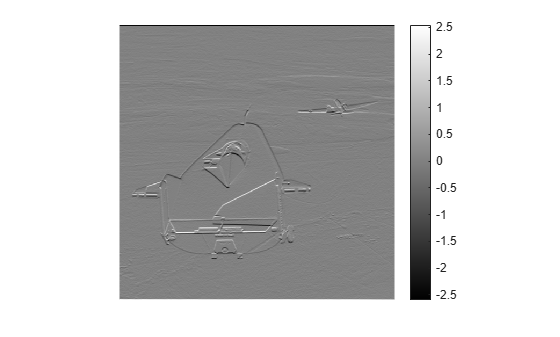# Add Color Bar to Displayed Grayscale Image

This example shows how to display a grayscale image with a color bar that indicates the mapping of data values to colors. Seeing the correspondence between data values and the colors displayed by using a color bar is especially useful if you are displaying unconventional range data as an image.

Read and display a grayscale image.

`I = imread('liftingbody.png');`

Convert the image to data type `double`. Data is in the range [0, 1].

```I = im2double(I); dataRangeI = [min(I(:)) max(I(:))]```
```dataRangeI = 1×2 0 1 ```

Filter the image using an edge detection filter. The filtered data exceeds the default range [0, 1] because the filter is not normalized.

```h = [1 2 1; 0 0 0; -1 -2 -1]; J = imfilter(I,h); dataRangeJ = [min(J(:)) max(J(:))]```
```dataRangeJ = 1×2 -2.5961 2.5451 ```

Display the filtered image using the full display range of the filtered data. `imshow` displays the minimum data value as black and the maximum data value as white.

`imshow(J,[])`

Use the `colorbar` function to add the color bar to the image.

`colorbar`